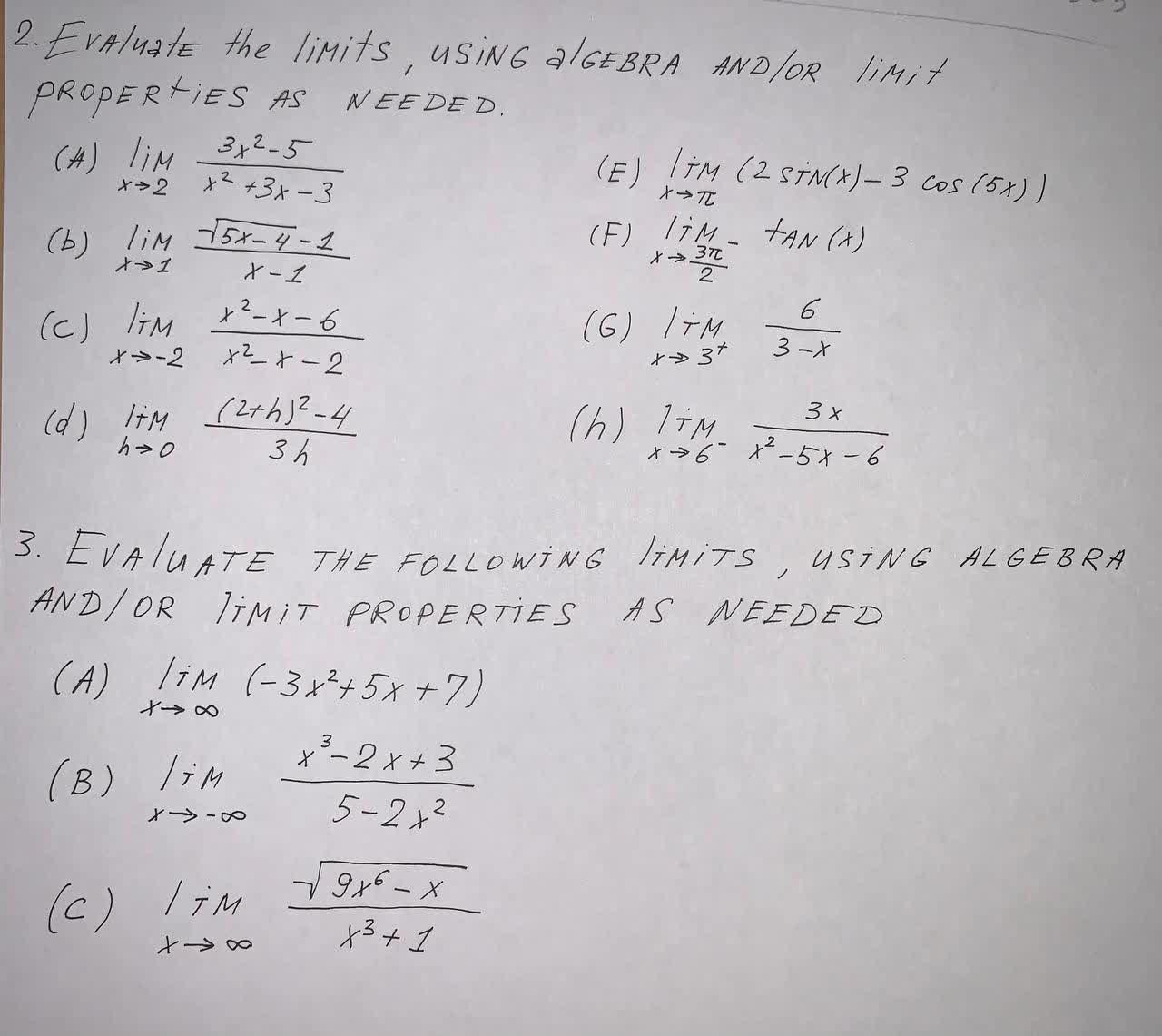# Get help with differential calculus problemsRecent questions in Differential Calculus
Limits and continuity
ANSWERED### Evaluate the following limit using the substitution $$\displaystyle{t}={x}^{{{6}}}$$ $$\displaystyle\lim_{{{t}\rightarrow{1}}}{b}{i}{g}{g{{\left({\frac{{\sqrt{{{3}}}{\left\lbrace{t}\right\rbrace}-{1}}}{{\sqrt{{{t}}}-{1}}}}{b}{i}{g}{g}\right)}}}$$

Analyzing functions
ANSWERED### Find the integer a such that $$\displaystyle{a}\equiv{99}{\left({b}\text{mod}{41}\right)}\ {\quad\text{and}\quad}\ {100}\leq{a}\leq{140}$$

Derivatives
ANSWERED### Find the derivative of $$\displaystyle{f{{\left({x}\right)}}}={e}^{{-{3}{x}}}$$

Analyzing functions
ANSWERED### Find the integer a such that $$\displaystyle{a}\equiv-{15}{\left({b}\text{mod}{27}\right)}\ {\quad\text{and}\quad}\ -{26}\leq{a}\leq{0}$$

Limits and continuity
ANSWERED### Find the following limits 1) $$\displaystyle\lim_{{{x}\to{0}}}\frac{{\tan{{\left({4}{x}\right)}}}}{{{x}+{\sin{{\left({2}{x}\right)}}}}}$$ 2) $$\displaystyle\lim_{{{x}\to\infty}}{\left({e}^{{{2}{x}}}+{x}\right)}^{{\frac{{1}}{{x}}}}$$

Analyzing functions
ANSWERED### Find the integer a such that $$\displaystyle{a}\equiv{24}{\left({b}\text{mod}{31}\right)}\ {\quad\text{and}\quad}\ -{15}\leq{a}\leq{15}$$

Analyzing functions
ANSWERED### If f(x) = 4x - 3, show that f(2x) = 2f(x) + 3

Limits and continuity
ANSWERED### Evaluate the limits, using algebra and/or limit properties as needed. $$\displaystyle\lim_{{{x}\rightarrow{2}}}{\frac{{{3}{x}^{{2}}-{5}}}{{{x}^{{2}}+{3}{x}-{3}}}}$$Derivatives
ANSWERED### Find the derivatives of the functions. $$\displaystyle{t}{\left({x}\right)}={4}^{{-{x}+{5}}}$$

Derivatives
ANSWERED### Find the derivatives of the functions. $$\displaystyle{s}{\left({x}\right)}={\ln{\sqrt{{{\ln{{x}}}}}}}$$

Derivatives
ANSWERED### Find the derivatives of the functions. $$\displaystyle{h}{\left({x}\right)}={\ln{{\left({\frac{{{y}_{{{x}}}}}{{{4}{x}-{2}}}}\right)}}}$$

Derivatives
ANSWERED### Find derivatives of the functions defined as follows. $$\displaystyle{y}=-{4}{e}^{{-{0.3}{x}}}$$

Derivatives
ANSWERED### Find the derivatives of the functions. $$\displaystyle{f{{\left({x}\right)}}}={\left({\ln}{\left|{x}\right|}\right)}^{{{2}}}$$

Derivatives
ANSWERED### Find the derivatives of y with respect to the appropriate variable. $$\displaystyle{y}={x}{{\sin}^{{-{1}}}{x}}+\sqrt{{{1}}}={x}^{{{2}}}$$

Derivatives
ANSWERED### Find derivatives for the functions. Assume a, b, c, and k are constants. $$\displaystyle{g{{\left({x}\right)}}}={2}{x}-{\frac{{{1}}}{{\sqrt{{{3}}}{\left\lbrace{x}\right\rbrace}}}}+{3}^{{{x}}}-{e}$$

Derivatives
ANSWERED### Find the derivatives of the given functions. $$\displaystyle{h}{\left({x}\right)}={2}{\sec{{x}}}+{3}{\tan{{x}}}+{3}{x}$$

Derivatives
ANSWERED### Find derivatives of the functions defined as follows. $$\displaystyle{y}={e}^{{{4}{x}}}$$

Derivatives
ANSWERED### Find derivatives for the functions. Assume a, b, c, and k are constants. $$y=17x+24x^{1x2F2}$$

Limits and continuity
ANSWERED### Find the limit (if it exists) and discuss the continuity of the function. $$\displaystyle\lim_{{{x},{y}}}\rightarrow{\left({1},{1}\right)}{\frac{{{x}{y}}}{{{x}²+{y}²}}}$$
ANSWERED